# 在Python中使用K-Means聚类和PCA主成分分析进行图像压缩

``````# image processing
from PIL import Image
from io import BytesIO
import webcolors

# data analysis
import math
import numpy as np
import pandas as pd

# visualization
import matplotlib.pyplot as plt
from importlib import reload
from mpl_toolkits import mplot3d
import seaborn as sns

# modeling
from sklearn.cluster import KMeans
from sklearn.decomposition import PCA
from sklearn.preprocessing import MinMaxScaler``````

## 探索图像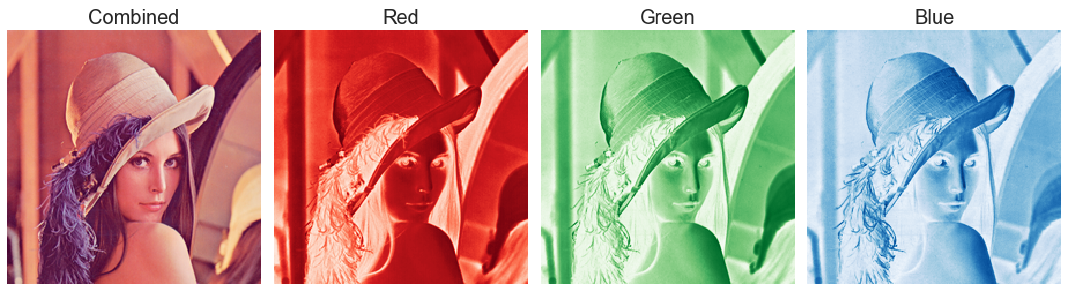``````ori_img = Image.open("images/lena.png")
ori_img````````````X = np.array(ori_img.getdata())
ori_pixels = X.reshape(*ori_img.size, -1)
ori_pixels.shape``````

``````def imageByteSize(img):
img_file = BytesIO()
image = Image.fromarray(np.uint8(img))
image.save(img_file, 'png')
return img_file.tell()/1024
ori_img_size = imageByteSize(ori_img)
ori_img_n_colors = len(set(ori_img.getdata()))
``````

lena.png的原始图像大小为86 KB，并具有37270种独特的颜色。 因此，我们可以说lena.png中的两个像素具有相同的精确RGB值的可能性很小。``ori_img_total_variance = sum(np.linalg.norm(X - np.mean(X, axis = 0), axis = 1)**2)``

## k-means聚类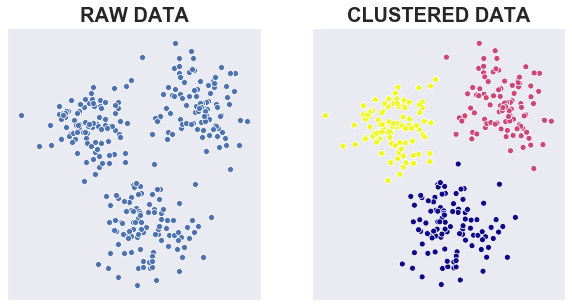### 算法

k-means聚类是一种常用的无监督学习算法，用于将数据集划分为k个聚类中心，其中k必须由用户预先指定。 该算法的目标是将现有数据点分类为几个集群，以便：

1. 同一集群中的数据尽可能相似
2. 来自不同集群的数据尽可能不同

1. 用户指定集群数k
2. 从数据集中随机选择k个不同的点作为初始聚类中心
3. 将每个数据点分配给最近的聚类中心，通常使用欧几里得距离
4. 通过取属于该集群的所有数据点的平均值来计算新聚类中心
5. 重复步骤3和4，直到收敛为止，即聚类中心位置不变

### 理念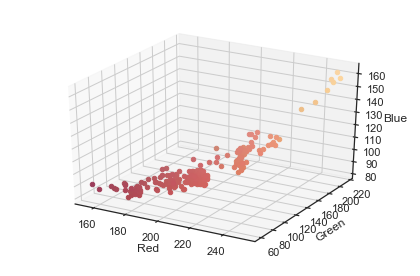### 简单的例子

``````kmeans = KMeans(n_clusters = 2,
n_jobs = -1,
random_state = 123).fit(X)
kmeans_df = pd.DataFrame(kmeans.cluster_centers_, columns = ['Red', 'Green', 'Blue'])``````

``````def closest_colour(requested_colour):
min_colours = {}
for key, name in webcolors.CSS3_HEX_TO_NAMES.items():
r_c, g_c, b_c = webcolors.hex_to_rgb(key)
rd = (r_c - requested_colour) ** 2
gd = (g_c - requested_colour) ** 2
bd = (b_c - requested_colour) ** 2
min_colours[(rd + gd + bd)] = name
return min_colours[min(min_colours.keys())]
def get_colour_name(requested_colour):
try:
closest_name = actual_name = webcolors.rgb_to_name(requested_colour)
except ValueError:
closest_name = closest_colour(requested_colour)
return closest_name
kmeans_df["Color Name"] = list(map(get_colour_name, np.uint8(kmeans.cluster_centers_)))
kmeans_df``````

``````def replaceWithCentroid(kmeans):
new_pixels = []
for label in kmeans.labels_:
pixel_as_centroid = list(kmeans.cluster_centers_[label])
new_pixels.append(pixel_as_centroid)
new_pixels = np.array(new_pixels).reshape(*ori_img.size, -1)
return new_pixels
new_pixels = replaceWithCentroid(kmeans)``````

``````def plotImage(img_array, size):
plt.imshow(np.array(img_array/255).reshape(*size))
plt.axis('off')
return plt

plotImage(new_pixels, new_pixels.shape).show()``````K-Means仅使用两种颜色成功地保留了lena.png的形状。 在视觉上，我们可以比较原始图像相似与压缩图像是否相似。 但是，我们如何用程序做到这一点？ 让我们介绍一组评估压缩图像的指标：

1. 在群集平方和（WCSS）中，测量群集中所有点与其群集中心的欧几里德距离平方的总和。

2. 在群集的平方和（BCSS）之间，测量所有聚类中心之间的欧几里得距离平方的总和。

3. 解释方差，衡量压缩图像效果可以通过压缩图像解释原始图像的百分比。 如果将每个像素视为一个单独的群集，则WCSS等于0。因此，Exparined Variance = 100％。

4. 图像大小，以千字节为单位，以评估缩小/压缩性能。

``````   def calculateBCSS(X, kmeans):
_, label_counts = np.unique(kmeans.labels_, return_counts = True)
diff_cluster_sq = np.linalg.norm(kmeans.cluster_centers_ - np.mean(X, axis = 0), axis = 1)**2
return sum(label_counts * diff_cluster_sq)
WCSS = kmeans.inertia_
BCSS = calculateBCSS(X, kmeans)
exp_var = 100*BCSS/(WCSS + BCSS)
print("WCSS: {}".format(WCSS))
print("BCSS: {}".format(BCSS))
print("Explained Variance: {:.3f}%".format(exp_var))
print("Image Size: {:.3f} KB".format(imageByteSize(new_pixels)))``````

WCSS: 109260691.314189
BCSS: 193071692.34763986
Explained Variance: 63.861%
Image Size: 4.384 KB

### 重复试验

1. 执行k-means以获取每个像素的聚类中心和聚类标签
2. 将每个像素替换为其聚类中心。
3. 保存指标值以进行进一步优化：WCSS，BCSS，解释方差和图像大小
4. 用越来越多的颜色绘制压缩图像
``````range_k_clusters = (2, 21)
kmeans_result = []
for k in range(*range_k_clusters):
# CLUSTERING
kmeans = KMeans(n_clusters = k,
n_jobs = -1,
random_state = 123).fit(X)

# REPLACE PIXELS WITH ITS CENTROID
new_pixels = replaceWithCentroid(kmeans)

# EVALUATE
WCSS = kmeans.inertia_
BCSS = calculateBCSS(X, kmeans)
exp_var = 100*BCSS/(WCSS + BCSS)

metric = {
"No. of Colors": k,
"Centroids": list(map(get_colour_name, np.uint8(kmeans.cluster_centers_))),
"Pixels": new_pixels,
"WCSS": WCSS,
"BCSS": BCSS,
"Explained Variance": exp_var,
"Image Size (KB)": imageByteSize(new_pixels)
}

kmeans_result.append(metric)
kmeans_result = pd.DataFrame(kmeans_result).set_index("No. of Colors")
kmeans_result``````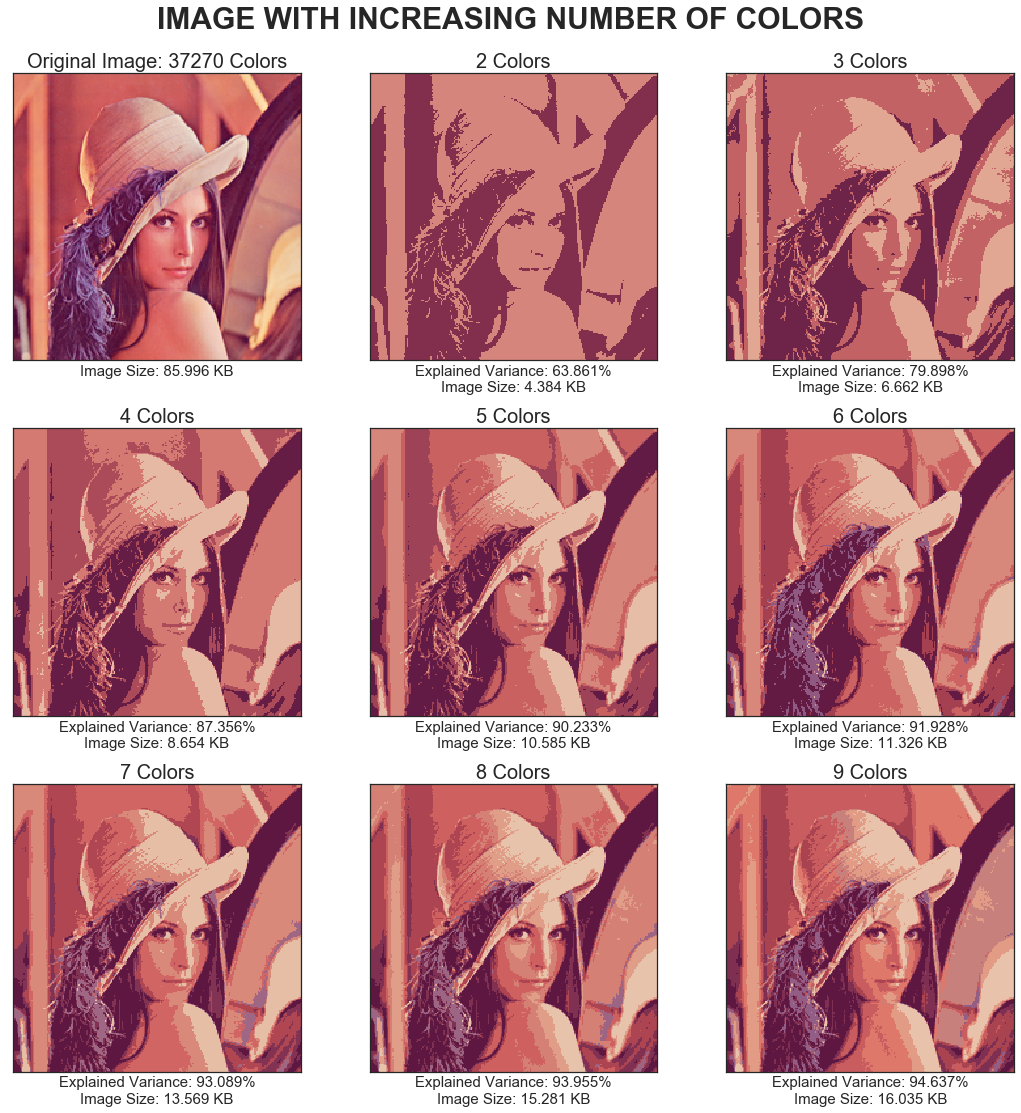))

### 聚类指标：最佳的颜色种类数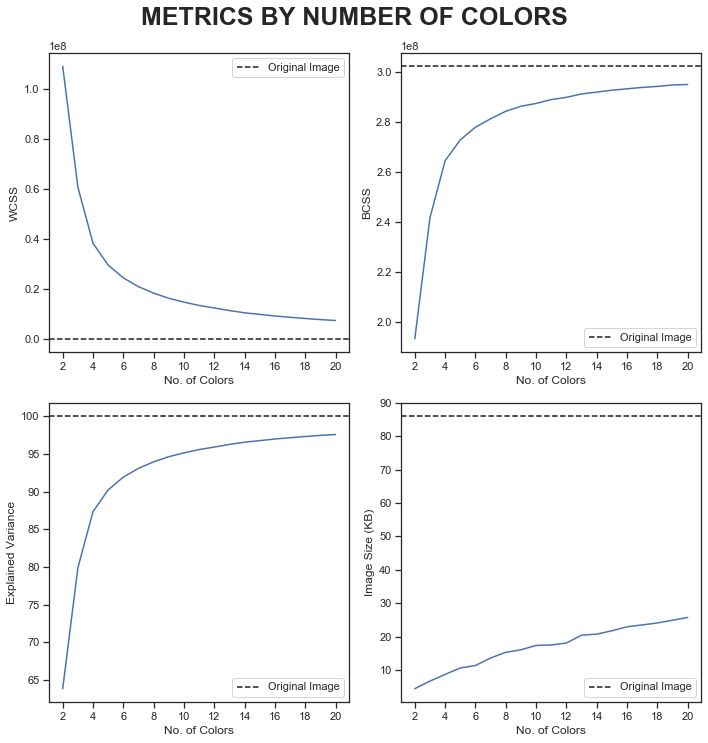1. 用直线连接曲线的第一个和最后一个点
2. 计算每个点到该线的垂直距离
3. 将距离最长的点视为拐点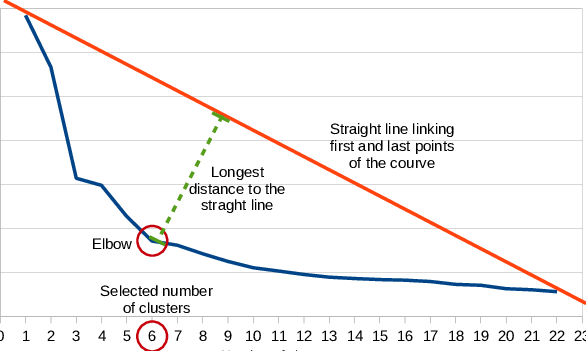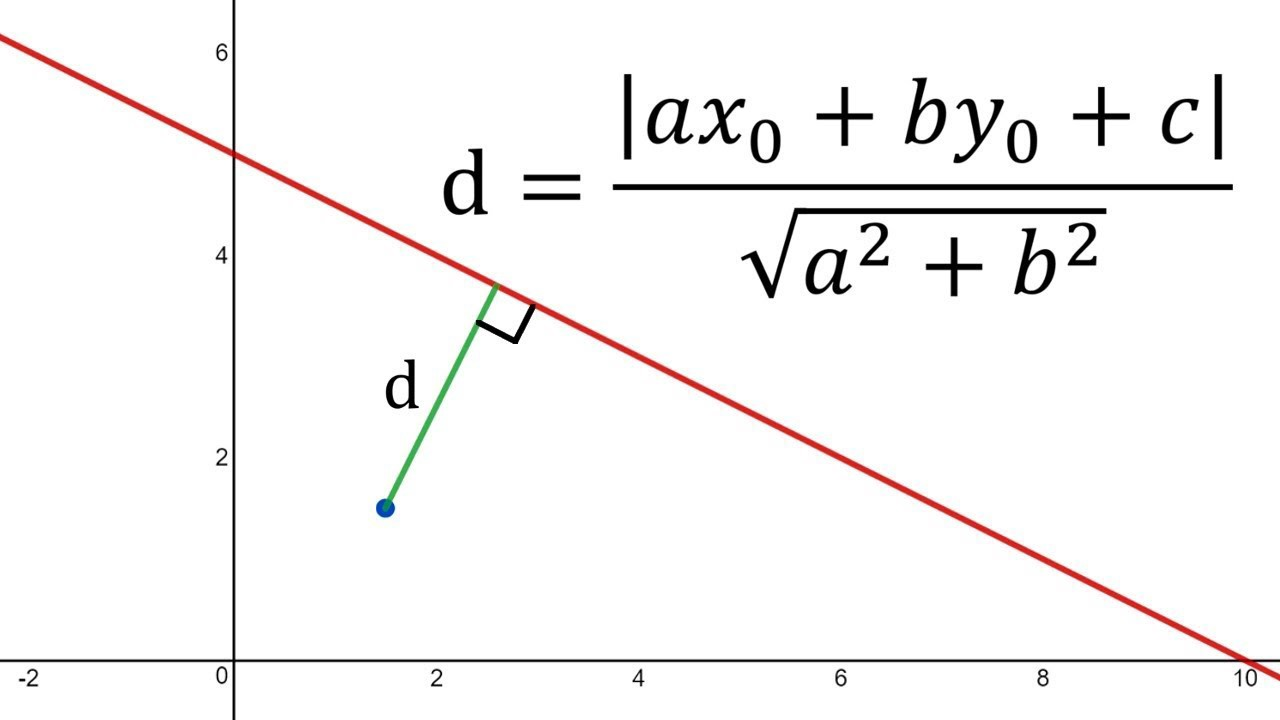``````def locateOptimalElbow(x, y):
# START AND FINAL POINTS
p1 = (x, y)
p2 = (x[-1], y[-1])

# EQUATION OF LINE: y = mx + c
m = (p2 - p1) / (p2 - p1)
c = (p2 - (m * p2))

# DISTANCE FROM EACH POINTS TO LINE mx - y + c = 0
a, b = m, -1
dist = np.array([abs(a*x0+b*y0+c)/math.sqrt(a**2+b**2) for x0, y0 in zip(x,y)])
return np.argmax(dist) + x``````

forward差异：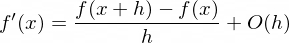backward差异：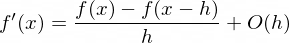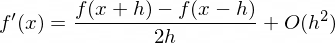f’（x）是函数f（x）的一阶导数
h是步长，在这种情况下，h = 1（颜色数的步长）
O（h）是一级误差项
O（h²）是二次误差项

``````def calculateDerivative(data):
derivative = []
for i in range(len(data)):
if i == 0:
# FORWARD DIFFERENCE
d = data[i+1] - data[i]
elif i == len(data) - 1:
# BACKWARD DIFFERENCE
d = data[i] - data[i-1]
else:
# CENTER DIFFERENCE
d = (data[i+1] - data[i-1])/2
derivative.append(d)
return np.array(derivative)
def locateDrasticChange(x, y):
# CALCULATE GRADIENT BY FIRST DERIVATIVE
first_derivative = calculateDerivative(np.array(y))

# CALCULATE CHANGE OF GRADIENT BY SECOND DERIVATIVE
second_derivative = calculateDerivative(first_derivative)
return np.argmax(np.abs(second_derivative)) + x``````

``````optimal_k = []
for col in kmeans_result.columns[2:]:
optimal_k_dict = {}
optimal_k_dict["Metric"] = col
if col == "Image Size (KB)":
optimal_k_dict["Method"] = "Derivative"
optimal_k_dict["Optimal k"] = locateDrasticChange(kmeans_result.index, kmeans_result[col].values)
else:
optimal_k_dict["Method"] = "Elbow"
optimal_k_dict["Optimal k"] = locateOptimalElbow(kmeans_result.index, kmeans_result[col].values)
optimal_k.append(optimal_k_dict)
optimal_k = pd.DataFrame(optimal_k)
optimal_k``````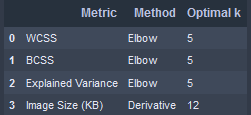### 与原始图像进行比较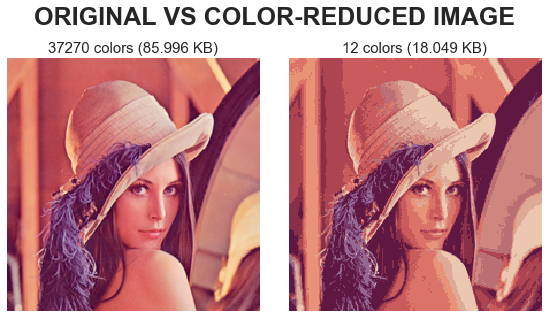``````relative_size = ori_vs_kmeans.loc["Color-Reduced", "Image Size (KB)"]/ori_vs_kmeans.loc["Original", "Image Size (KB)"]
print("Reduction: {:.3f}% from original image size".format((1-relative_size)*100))
print("Explained Variance: {:.3f}%".format(ori_vs_kmeans.loc["Color-Reduced", "Explained Variance"]))``````

## 主成分分析（PCA）

### 概念

PCA是用于降维的无监督学习技术之一。 它从协方差矩阵计算出特征向量，然后将其称为主轴，并按称为解释方差百分比的特征值进行递减排序。 然后将数据集居中并投影到形成主要成分（或分数）的主轴上。 为了减少数据维度，我们仅保留一定数量的主成分n来解释原始数据集的方差，而忽略其余部分。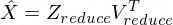Z_reduce的尺寸为m×n_select
V_reduce的维数为n×n_select
T是矩阵转置运算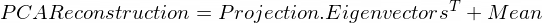### RGB通道的主要组件

``````res = []
cum_var = []
X_t = np.transpose(X)
for channel in range(3):
# SEPARATE EACH RGB CHANNEL
pixel = X_t[channel].reshape(*ori_pixels.shape[:2])

# PCA
pca = PCA(random_state = 123)
pixel_pca = pca.fit_transform(pixel)

pca_dict = {
"Projection": pixel_pca,
"Components": pca.components_,
"Mean": pca.mean_
}
res.append(pca_dict)

# EVALUATION
cum_var.append(np.cumsum(pca.explained_variance_ratio_))``````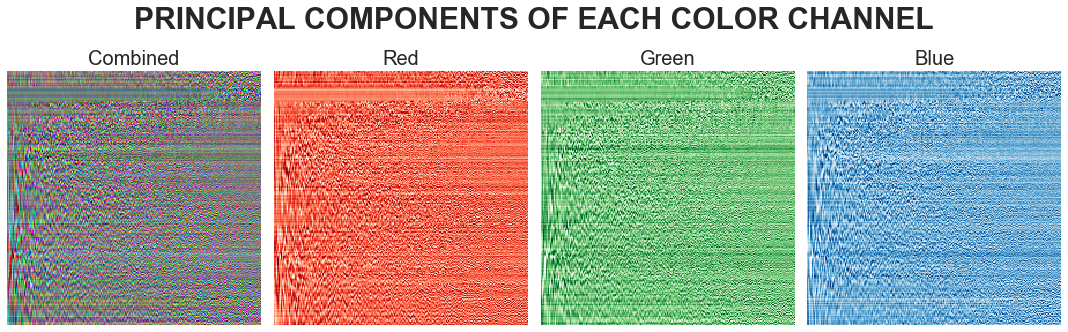PC的可视化信息不足，随机性很大。 我们应该引入一个称为解释方差的指标来评估PC性能。 取值范围是0到100％，表示原始图像和压缩图像之间的相似度。

``````cum_var_df = pd.DataFrame(np.array(cum_var).T * 100,
index = range(1, pca.n_components_+1),
columns = ["Explained Variance by Red",
"Explained Variance by Green",
"Explained Variance by Blue"])
cum_var_df["Explained Variance"] = cum_var_df.mean(axis = 1)
cum_var_df``````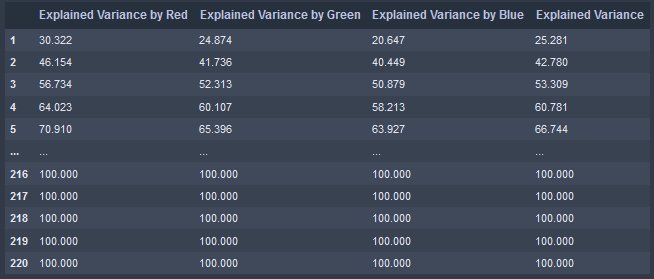### 重复试验

1. 区分PCA投影的前n_select列和组件的前n_select行
2. 使用PCA建立公式和原始图像
3. 对红色，绿色和蓝色每个颜色通道重复步骤1-2。
4. 将三种颜色通道的PCA重构组合为一个3D矩阵
5. 保存指标值(解释方差，图像大小和颜色数量)以进行进一步优化
6. 用越来越多的主成分绘制压缩（重构）图像
``````pca_results = []
for n in range(1, pca.n_components_+1):
# SELECT N-COMPONENTS FROM PC
temp_res = []
for channel in range(3):
pca_channel = res[channel]
pca_pixel = pca_channel["Projection"][:, :n]
pca_comp = pca_channel["Components"][:n, :]
pca_mean = pca_channel["Mean"]
compressed_pixel = np.dot(pca_pixel, pca_comp) + pca_mean
temp_res.append(compressed_pixel.T)
compressed_image = np.transpose(temp_res)

pca_dict = {
"n": n,
"Pixels": compressed_image,
"Explained Variance": cum_var_df["Explained Variance"][n],
"Image Size (KB)": imageByteSize(compressed_image),
"No. of Colors": len(np.unique(np.uint8(compressed_image).reshape(-1, 3), axis = 0))
}

pca_results.append(pca_dict)
pca_results = pd.DataFrame(pca_results).set_index("n")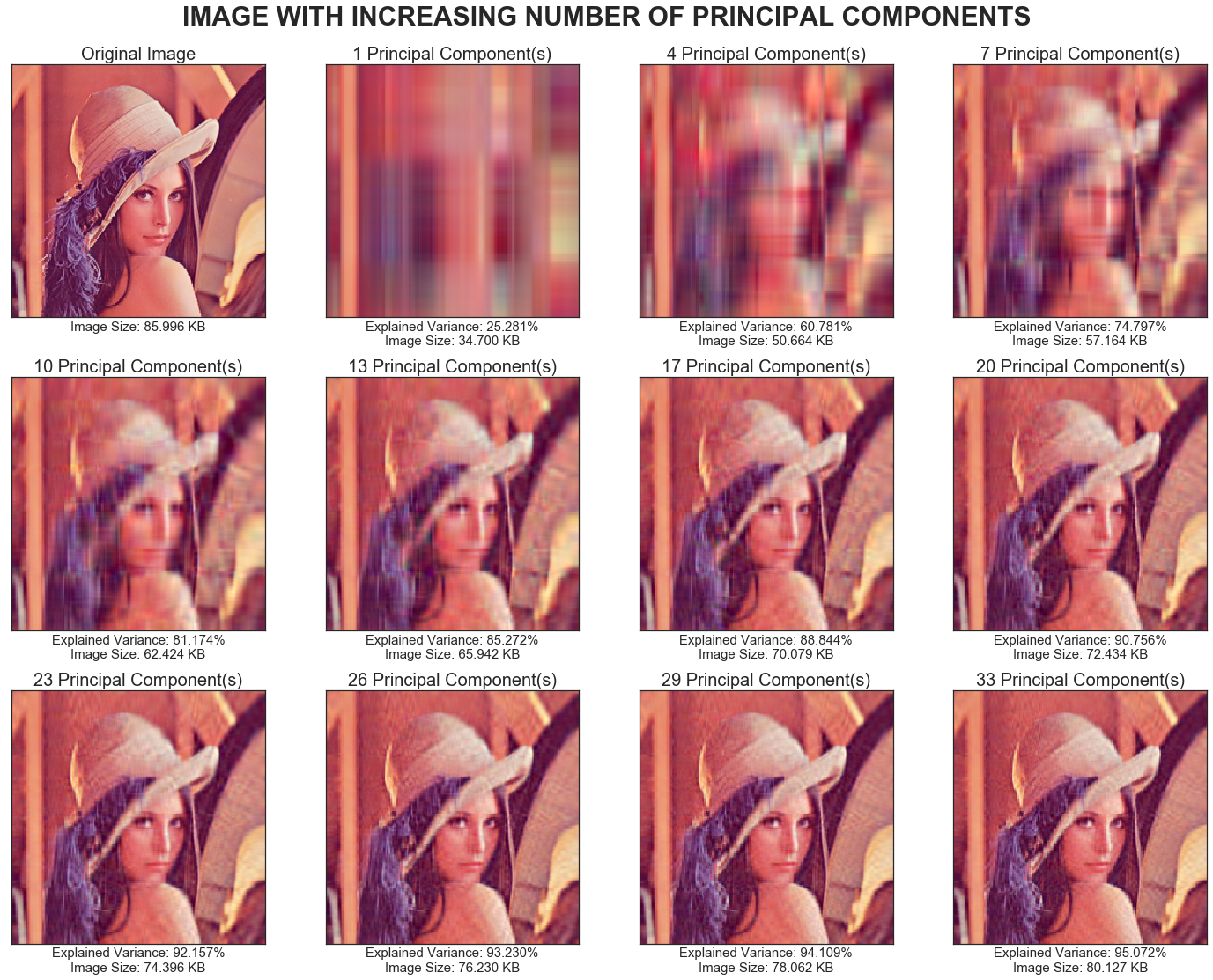### PCA指标：主成分的最佳数量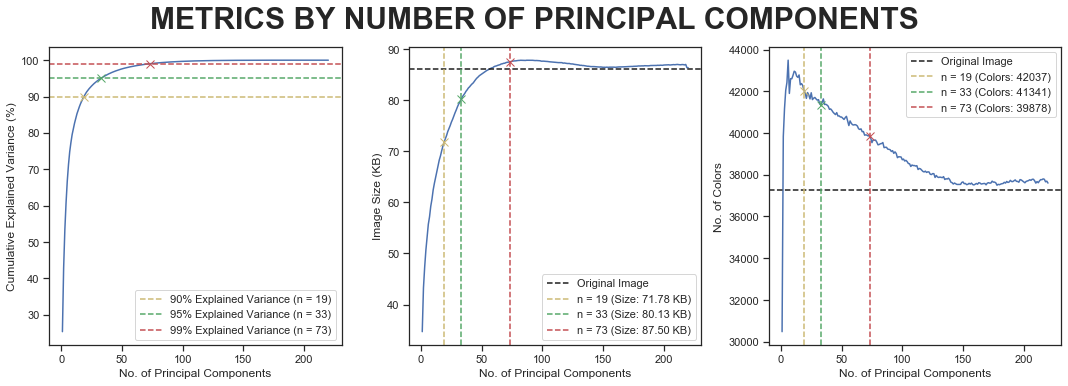### 与原始图像进行比较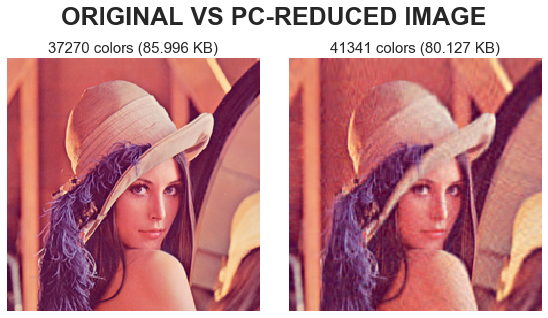``````relative_size = ori_vs_pca.loc["PC-Reduced", "Image Size (KB)"]/ori_vs_kmeans.loc["Original", "Image Size (KB)"]
print("Reduction: {:.3f}% from original image size".format((1-relative_size)*100))
print("Explained Variance: {:.3f}%".format(ori_vs_pca.loc["PC-Reduced", "Explained Variance"]))``````

## k-means和PCA的比较

1. 图片大小（以千字节为单位）
2. 解释方差
3. 图像中存在的颜色数
``````reduction_kmeans = (1-final_compare.loc["Color-Reduced", "Image Size (KB)"] / ori_img_size) * 100
reduction_pca = (1-final_compare.loc["PC-Reduced", "Image Size (KB)"] / ori_img_size) * 100
print("Image Size Reduction using K-Means: {:.3f}%".format(reduction_kmeans))
print("Image Size Reduction using PCA: {:.3f}%".format(reduction_pca))``````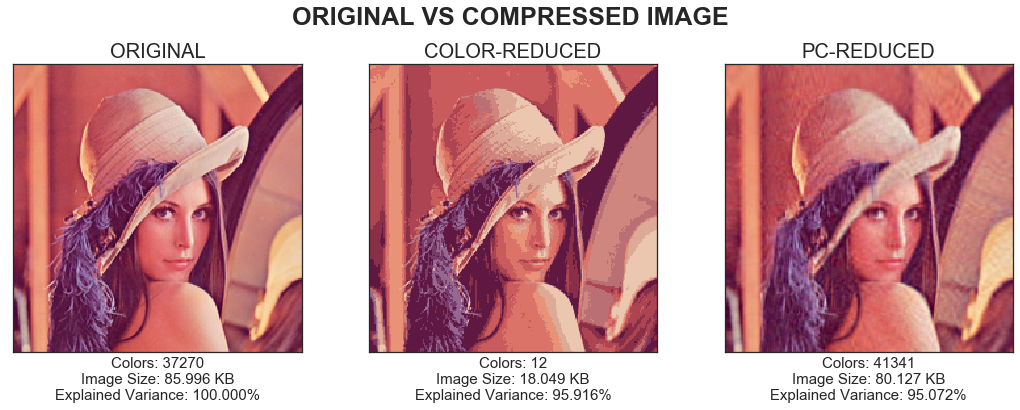## 结论

1. 使用最长垂直距离的方法
2. 使用有限差分法和二阶导数

deephub翻译组：孟翔杰

###### 评论